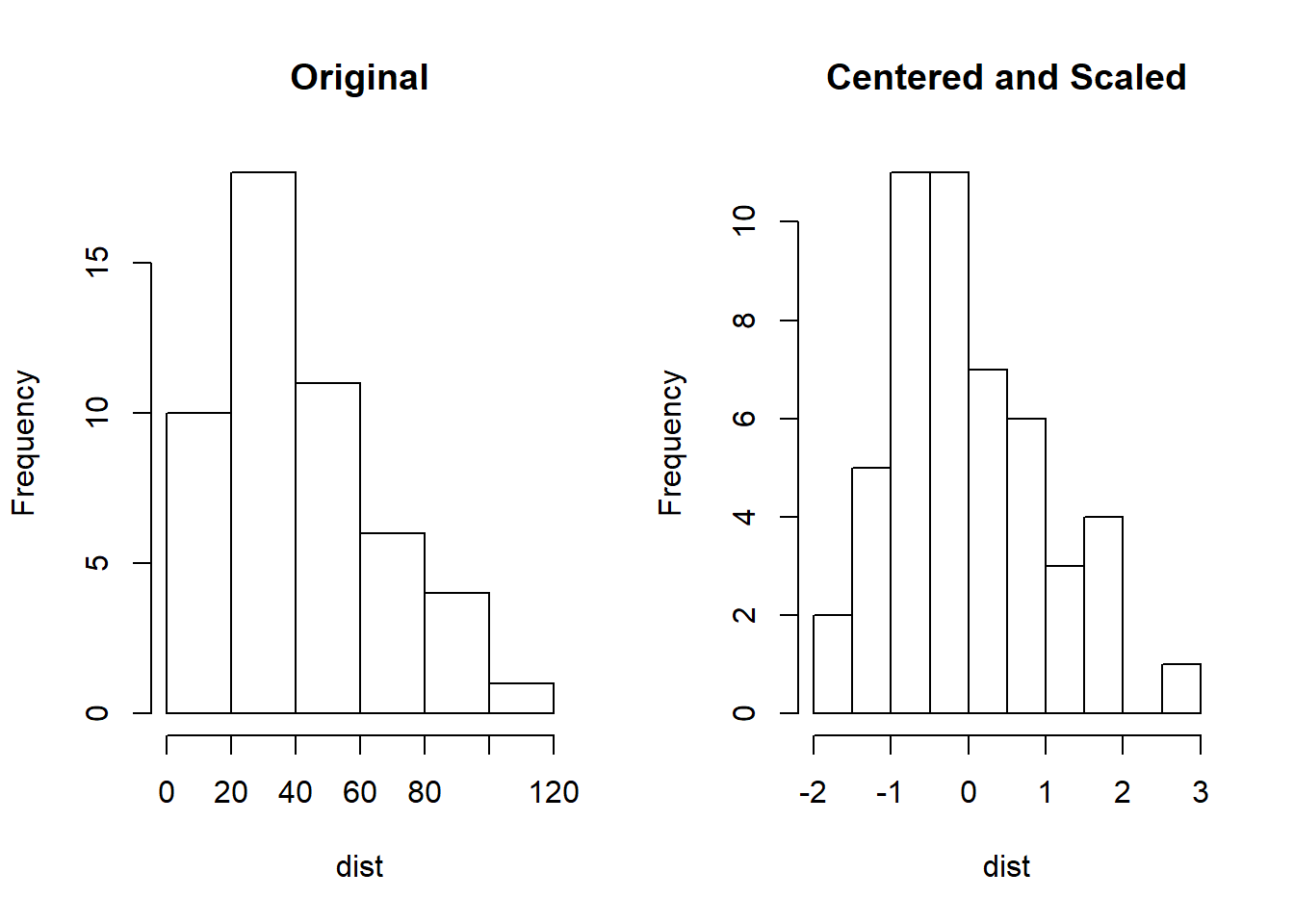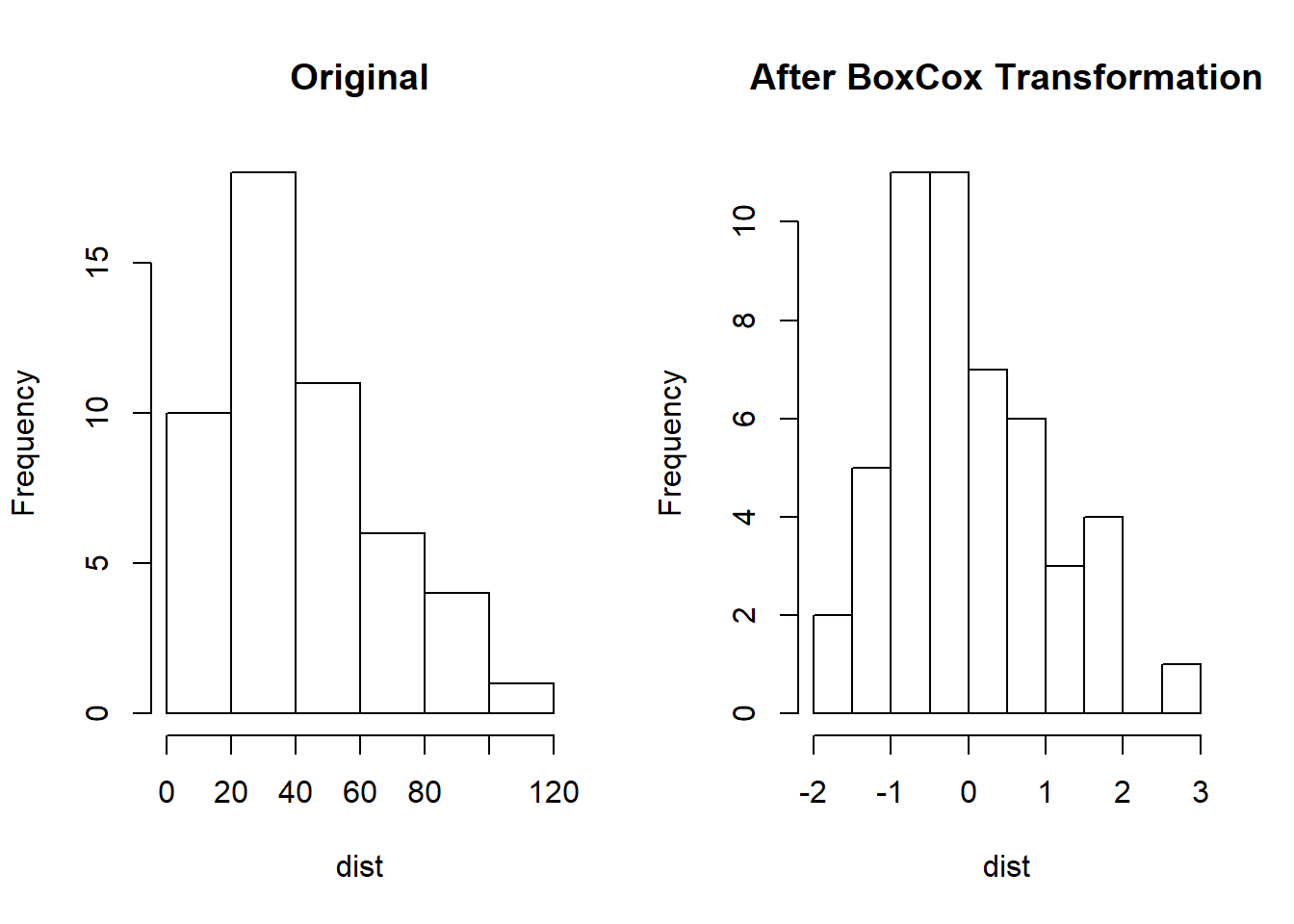I have already covered scling and centering but I wanted to take this a bit deeper this time. I aslo want to dive deeper into skew.

## Centering and Scaling

It is the most straightforward data transformation. It centers and scales a variable to mean 0 and standard deviation 1. It ensures that the criterion for finding linear combinations of the predictors is based on how much variation they explain and therefore improves the numerical stability.

head(cars)
##   speed dist
## 1     4    2
## 2     4   10
## 3     7    4
## 4     7   22
## 5     8   16
## 6     9   10
trans<-preProcess(cars, method=c("center","scale"))
transformed <- predict(trans,cars)
{par(mfrow=c(1, 2))
hist(cars$dist, main="Original", xlab="dist") hist(transformed$dist, main="Centered and Scaled", xlab="dist")}Sometimes you only need to scale the variable. For example, if the model adds penalty to the parameter estimates (such as L2 penalty is ridge regression and L1 penalty in LASSO), the variables need to have similar scale to ensure a fair variable selection.

qscale<-function(dat){
for (i in 1:ncol(dat)){
up <- quantile(dat[,i], 0.99)
low <- quantile(dat[,i], 0.01)
diff <- up-low
dat[,i] <- (dat[, i]-low)/diff
}
return(dat)
}

Note: 99% and 1% quantile are used instead of maximum and minimum values to resist the impact of outliers.

In order to illustrate, let’s simulate a data set with three variables: income, age and education. (This might look familar since I have used this fake data before).

#Make up some data
income <- round(runif(100, min = 35000, max = 350000), 0)
age <- round(runif(100, min=18, max=72), 0)
myData <- data.frame(age, income)
noise <- round(runif(100, min = 1500, max = 15000), 0)
myData$income <- myData$income + noise
myData <- arrange(myData, desc(income))
myDataeducation <- as.factor(sample(c("High School", "Bachelors", "Masters", "Doctorate"), 100, replace = TRUE, prob =c(0.7, 0.15, 0.12, 0.03) )) summary(myData[,c("income","age")]) ## income age ## Min. : 48960 Min. :18.00 ## 1st Qu.:131982 1st Qu.:30.00 ## Median :211606 Median :45.00 ## Mean :207488 Mean :45.50 ## 3rd Qu.:278594 3rd Qu.:62.25 ## Max. :357325 Max. :71.00 Clearly income and age are not on the same scale.Apply the function qscale() on the data. myNewData <- qscale(myData[, c("income", "age")]) summary(myNewData) ## income age ## Min. :-0.004211 Min. :-0.01903 ## 1st Qu.: 0.270426 1st Qu.: 0.21169 ## Median : 0.533822 Median : 0.50010 ## Mean : 0.520198 Mean : 0.50971 ## 3rd Qu.: 0.755417 3rd Qu.: 0.83176 ## Max. : 1.015860 Max. : 1.00000 Now the scales of income and age are aligned (and remember extreme outliers have been removed). ## Resolve Skewness Skewness is defined to be the third standardized central moment. Does that help? (It does for the math whizzes.) You can easily tell if a distribution is skewed by simple visualization. There are different ways may help to remove skewness such as log, square root or inverse. However it is often difficult to determine from plots which transformation is most appropriate for correcting skewness. The Box-Cox procedure automatically identified a transformation from the family of power transformations that are indexed by a parameter λ. This family includes: • log transformation (λ=0) • square transformation (λ=2) • square root (λ=0.5) • inverse (λ=−1) • others in-between Use preProcess() in caret to apply this transformation by changing the method argument. mySkew1 <- preProcess(cars, method = c("BoxCox")) mySkew1 ## Created from 50 samples and 2 variables ## ## Pre-processing: ## - Box-Cox transformation (2) ## - ignored (0) ## ## Lambda estimates for Box-Cox transformation: ## 1, 0.5 The output shows the sample size (50), number of variables (2) and the λ estimates for each variable. After calling preProcess(), predict() method applies the results to a data frame. myTransformed <- predict(mySkew1, cars) {par(mfrow=c(1,2)) hist(carsdist, main="Original", xlab="dist")
hist(transformed$dist, main="After BoxCox Transformation", xlab="dist")}An alternative is to use BoxCoxTrans() in caret. Is it a good thing or a bad thing to have multiple ways to get to the same result? I prefer to pick one and stick to it. In this case, I prefer BoxCoxTrans(). It is just easier to remember. You can use function skewness() in package e1071 to get the skewness statistics. myBoxCoxTrans <- BoxCoxTrans(cars$dist)
myBoxCoxTrans
## Box-Cox Transformation
##
## 50 data points used to estimate Lambda
##
## Input data summary:
##    Min. 1st Qu.  Median    Mean 3rd Qu.    Max.
##    2.00   26.00   36.00   42.98   56.00  120.00
##
## Largest/Smallest: 60
## Sample Skewness: 0.759
##
## Estimated Lambda: 0.5
myTransformed2 <- predict(myBoxCoxTrans, cars\$dist)
skewness(myTransformed2)#required e1071
##  -0.01902765

The estimated λ is the same 0.5. Original skewness is 0.759 and after transformation, the skewness is -0.01902765 which is close to 0.